# 光线跟踪之 ：Phong模型，镜面反射及阴影

Introduction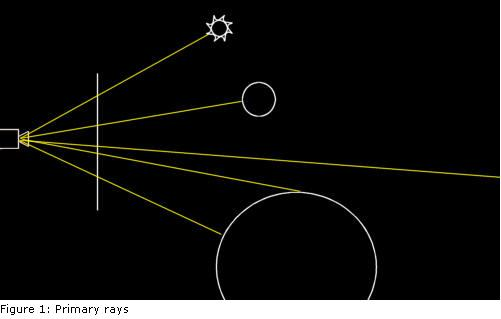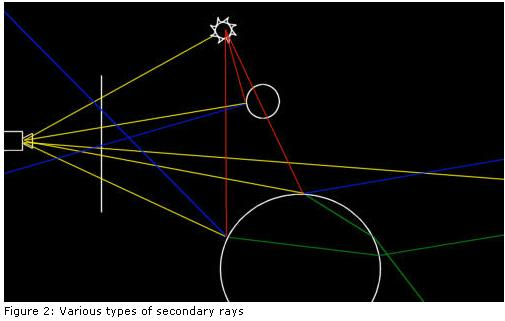# Reflections

            // calculate reflection
float refl = prim->GetMaterial()->GetReflection();
if (refl > 0.0f)
{//几何体材质的反射度大于
vector3 N = prim->GetNormal( pi );//几何体的法向量
vector3 R = a_Ray.GetDirection() - 2.0f * DOT( a_Ray.GetDirection(), N ) * N;
if (a_Depth < TRACEDEPTH)
{//层数还没到上限
Color rcol( 0, 0, 0 );
float dist;
Raytrace( Ray( pi + R * EPSILON, R ), rcol, a_Depth + 1, a_RIndex, dist );
a_Acc += refl * rcol * prim->GetMaterial()->GetColor();//颜色值中加入反射光的贡献值
}
}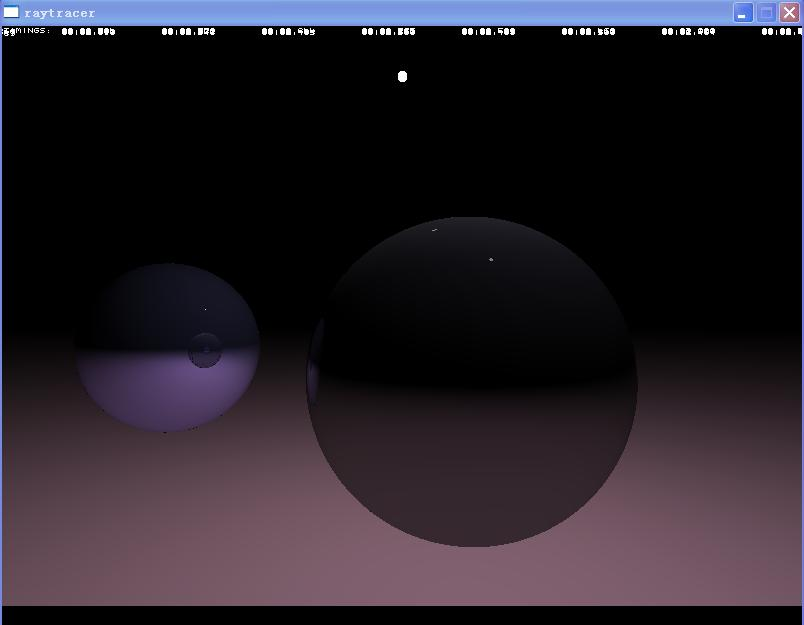# Phong光照模型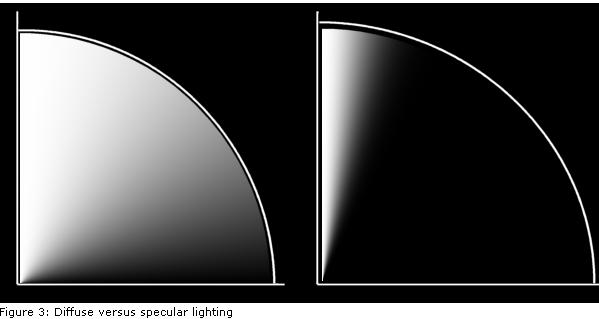intensity = diffuse * (L.N) + specular * (V.R)n

(这里L是从相交点到光源的向量，N是平面法向量，V是视线方向,R是L在表面上的反射向量)。注意这个公式包含了散射和镜面反射光。

vector3 V = a_Ray.GetDirection();//光线方向
vector3 R = L - 2.0f * DOT( L, N ) * N;

float dot = DOT( V, R );
if (dot > 0)
{
float spec = powf( dot, 20 ) * prim->GetMaterial()->GetSpecular() * shade;
// add specular component to ray color
a_Acc += spec * light->GetMaterial()->GetColor();
}增加了Phong光照模型的计算后，产生的结果如下图：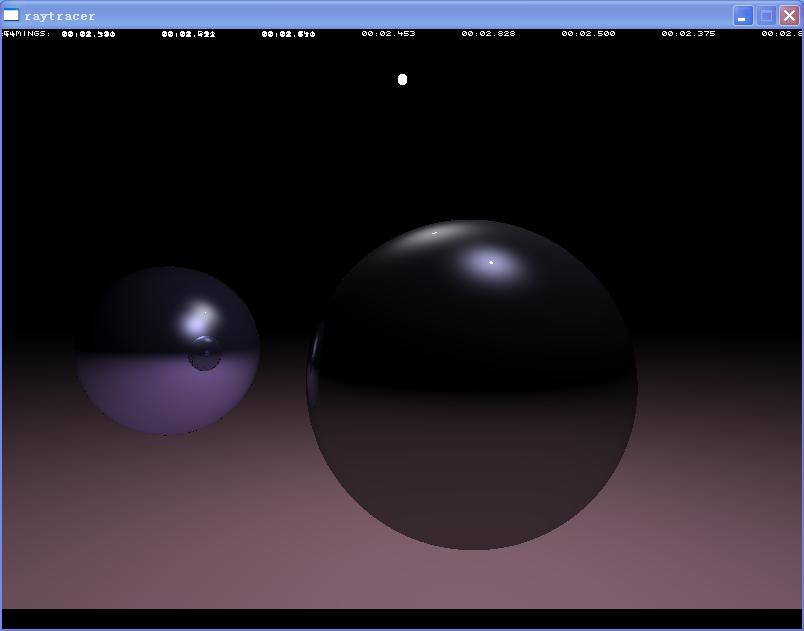Shadows最后一种类型的secondary ray是阴影线。这种光线和其他的不同：它对于产生它的光线的颜色没有贡献；相反，它们经常用来判段一个光源是否可以“看见”一个相交点。// handle point light source
if (light->GetType() == Primitive::SPHERE)
{
vector3 L = ((Sphere*)light)->GetCentre() - pi;
float tdist = LENGTH( L );
L *= (1.0f / tdist);
Ray r = Ray( pi + L * EPSILON, L );
for ( int s = 0; s < m_Scene->GetNrPrimitives(); s++ )
{
Primitive* pr = m_Scene->GetPrimitive( s );
if ((pr != light) && (pr->Intersect( r, tdist )))
{
break;
}
}
}

这段代码做的事情应该很熟悉了吧。测试的结果保存在一个浮点数”shade’中：值为1代表一个可见的光源，为0则是一个要排除的光源。这里使用一个浮点数是有点奇怪，但到后面我们会增加面光源，而面光源一般是部分可见的。在那种情况下我们会使用值在0到1之间的’shade’。另外不得不提的是，上面这段代码并不一定可以找到阴影线与场景中的几何体之间最近的相交点。这点并不影响：只要能找到比光源近的几何体就可以了。这样做是出于优化的考虑，因为只要我们尽快找到一个相交点就马上终止循环了。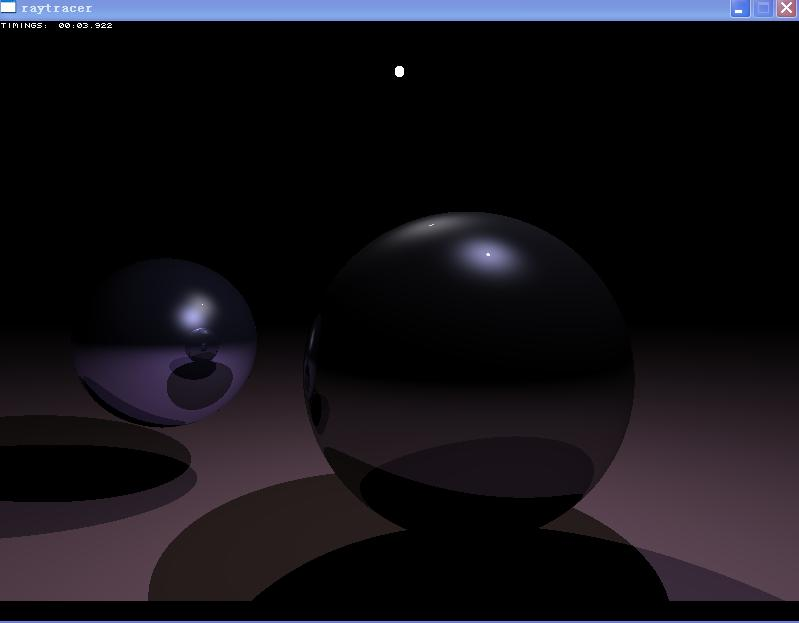上图就是我们这篇文章最后得到的效果了，两个光线跟踪的球体，带反射，散射，和镜面光照，还有来自两个光源产生的阴影。附带一句，这个光线跟踪器有一个bug：阴影测试的结果不仅用于计算散射因子，也用来计算镜面反射因子。严格来说，这是不对的，作者在后面的文章中会提出一个解决方案的。光线跟踪
Primitive* Engine::Raytrace( Ray& a_Ray, Color& a_Acc, int a_Depth, float a_RIndex, float& a_Dist )
{
if (a_Depth > TRACEDEPTH) return 0;
// trace primary ray
a_Dist = 1000000.0f;
vector3 pi;
Primitive* prim = 0;
int result;
// find the nearest intersection
for ( int s = 0; s < m_Scene->GetNrPrimitives(); s++ )
{
Primitive* pr = m_Scene->GetPrimitive( s );
int res;
if (res = pr->Intersect( a_Ray, a_Dist ))
{
prim = pr;
result = res; // 0 = miss, 1 = hit, -1 = hit from inside primitive
}
}
// no hit, terminate ray
if (!prim) return 0;
// handle intersection
if (prim->IsLight())
{
// we hit a light, stop tracing
a_Acc = Color( 1, 1, 1 );
}
else
{
// determine color at point of intersection
pi = a_Ray.GetOrigin() + a_Ray.GetDirection() * a_Dist;
// trace lights
for ( int l = 0; l < m_Scene->GetNrPrimitives(); l++ )
{
Primitive* p = m_Scene->GetPrimitive( l );
if (p->IsLight())
{
Primitive* light = p;
// handle point light source
if (light->GetType() == Primitive::SPHERE)
{
vector3 L = ((Sphere*)light)->GetCentre() - pi;
float tdist = LENGTH( L );
L *= (1.0f / tdist);
Ray r = Ray( pi + L * EPSILON, L );
for ( int s = 0; s < m_Scene->GetNrPrimitives(); s++ )
{
Primitive* pr = m_Scene->GetPrimitive( s );
if ((pr != light) && (pr->Intersect( r, tdist )))
{
break;
}
}
}
vector3 L = ((Sphere*)light)->GetCentre() - pi;
NORMALIZE( L );
vector3 N = prim->GetNormal( pi );
if (prim->GetMaterial()->GetDiffuse() > 0)
{
float dot = DOT( L, N );
if (dot > 0)
{
float diff = dot * prim->GetMaterial()->GetDiffuse() * shade;
// add diffuse component to ray color
a_Acc += diff * light->GetMaterial()->GetColor() * prim->GetMaterial()->GetColor();
}
}
// determine specular component
if (prim->GetMaterial()->GetSpecular() > 0)
{
// point light source: sample once for specular highlight
vector3 V = a_Ray.GetDirection();
vector3 R = L - 2.0f * DOT( L, N ) * N;
float dot = DOT( V, R );
if (dot > 0)
{
float spec = powf( dot, 20 ) * prim->GetMaterial()->GetSpecular() * shade;
// add specular component to ray color
a_Acc += spec * light->GetMaterial()->GetColor();
}
}
}
}
// calculate reflection
float refl = prim->GetMaterial()->GetReflection();
if (refl > 0.0f)
{//几何体材质的反射度大于0
vector3 N = prim->GetNormal( pi );//几何体的法向量
vector3 R = a_Ray.GetDirection() - 2.0f * DOT( a_Ray.GetDirection(), N ) * N;
if (a_Depth < TRACEDEPTH)
{//层数还没到上限
Color rcol( 0, 0, 0 );
float dist;
Raytrace( Ray( pi + R * EPSILON, R ), rcol, a_Depth + 1, a_RIndex, dist );
a_Acc += refl * rcol * prim->GetMaterial()->GetColor();//颜色值中加入反射光的贡献值
}
}
}
// return pointer to primitive hit by primary ray
return prim;
}

 原文链接: http://www.devmaster.net/articles/raytracing_series/part2.php

06-23
06-025794
06-021249
03-261255
10-21752
02-245885
09-12335
04-11462
04-261118
04-12110
08-091万+
02-091024
07-167953
11-231834

### “相关推荐”对你有帮助么？

•非常没帮助
•没帮助
•一般
•有帮助
•非常有帮助被折叠的  条评论 为什么被折叠?到【灌水乐园】发言点击重新获取扫码支付1.余额是钱包充值的虚拟货币，按照1:1的比例进行支付金额的抵扣。
2.余额无法直接购买下载，可以购买VIP、C币套餐、付费专栏及课程。余额充值